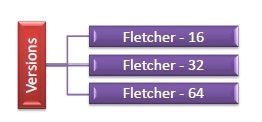# Fletcher’s Checksum

Fletcher checksum is an error – detection technique that uses two checksums to determine single-bit errors in a message transmitted over network channels. It is a block code technique that was devised by John G. Fletcher in 1970s at Lawrence Livermore Labs, USA.

The checksums are created based on the data values in the data blocks to be transmitted and appended to the data. When the receiver gets this data, the checksums are re-calculated and compared with the existing checksums. A non-match indicates an error.

The error-detection capabilities of this method is nearly same as that of Cyclic Redundancy Check (CRC) but requires much less computational effort.

## Versions of Fletcher’s Checksum

There are three popular algorithms of Fletcher’s checksum −• Fletcher – 16 − The data word is divided into 8-bit blocks. Then, two 8-bit checksums are computed and are appended to form a 16-bit Fletcher checksum.

• Fletcher – 32 − The data word is divided into 16-bit blocks. Two 16-bit checksums are computed and are appended to form a 32-bit Fletcher checksum.

• Fletcher – 64 − The data word is divided into 32-bit blocks. Two 32-bit checksums are computed and are appended to form a 32-bit Fletcher checksum.

## Algorithm for Computing Fletcher’s Checksum

INPUT : data blocks of equal sizes, 𝑏1,𝑏2………… 𝑏𝑛
OUTPUT : two checksums, 𝑐ℎ𝑒𝑐𝑘𝑠𝑢𝑚1 and 𝑐ℎ𝑒𝑐𝑘𝑠𝑢𝑚2, of 1 byte each

Step 1) Initialize partial sums, and sums, 𝑐1=0 and 𝑐2=0

Step 2) For each data block, 𝑏𝑖

• i. Add 𝑏𝑖 to 𝑐1

Step 3) Compute checksums,

• 𝑐ℎ𝑒𝑐𝑘𝑠𝑢𝑚1= 𝑐𝑀𝑂𝐷 256 and 𝑐ℎ𝑒𝑐𝑘𝑠𝑢𝑚2= 𝑐𝑀𝑂𝐷 256

Step 4) Append checksums, 𝑐ℎ𝑒𝑐𝑘𝑠𝑢𝑚1 and 𝑐ℎ𝑒𝑐𝑘𝑠𝑢𝑚2, to the data blocks, 𝑏1,𝑏2………… 𝑏𝑛

## Example of Computation of Fletcher’s Checksum

Let there be five data blocks, 163, 200, 19, 74 and 88. The computations are −

Block Number    Data Block   c1    c2
-              -        0     0
1             163      163   163
2             200      363   526
3              19      382 908
4 74 456 1364
5 88 544 1908

𝑐ℎ𝑒𝑐𝑘𝑠𝑢𝑚= 544 𝑀𝑂𝐷 256 = 34

𝑐ℎ𝑒𝑐𝑘𝑠𝑢𝑚= 1908 𝑀𝑂𝐷 256 = 123